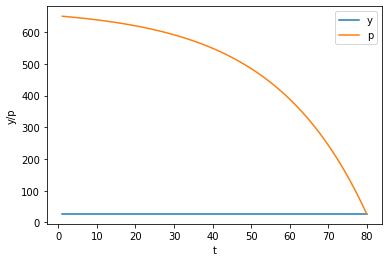# Univariate Time Series with Matrix Algebra¶

## Overview¶

This lecture uses matrices to solve some linear difference equations.

As a running example, we’ll study a second-order linear difference equation that was the key technical tool in Paul Samuelson’s 1939 article [Sam39] that introduced the multiplier-accelerator model.

This model became the workhorse that powered early econometric versions of Keynesian macroeconomic models in the United States.

You can read about the details of that model in this QuantEcon lecture.

(That lecture also describes some technicalities about second-order linear difference equations.)

We’ll also study a “perfect foresight” model of stock prices that involves solving a “forward-looking” linear difference equation.

We will use the following imports:

In :
import numpy as np
import matplotlib.pyplot as plt
%matplotlib inline


## Samuelson’s model¶

Let $t = 0, \pm 1, \pm 2, \ldots$ index time.

For $t = 1, 2, 3, \ldots, T$ suppose that

$$y_{t} = \alpha_{0} + \alpha_{1} y_{t-1} + \alpha_{2} y_{t-2} \tag{1}$$

where we assume that $y_0$ and $y_{-1}$ are given numbers that we take as initial conditions.

In Samuelson’s model, $y_t$ stood for national income or perhaps a different measure of aggregate activity called gross domestic product (GDP) at time $t$.

Equation (1) is called a second-order linear difference equation.

But actually, it is a collection of $T$ simultaneous linear equations in the $T$ variables $y_1, y_2, \ldots, y_T$.

Note: To be able to solve a second-order linear difference equations, we require two boundary conditions that can take the form either of two initial conditions or two terminal conditions or possibly one of each.

Let’s write our equations as a stacked system

$$\underset{\equiv A}{\underbrace{\left[\begin{array}{cccccccc} 1 & 0 & 0 & 0 & \cdots & 0 & 0 & 0\\ -\alpha_{1} & 1 & 0 & 0 & \cdots & 0 & 0 & 0\\ -\alpha_{2} & -\alpha_{1} & 1 & 0 & \cdots & 0 & 0 & 0\\ 0 & -\alpha_{2} & -\alpha_{1} & 1 & \cdots & 0 & 0 & 0\\ \vdots & \vdots & \vdots & \vdots & \cdots & \vdots & \vdots & \vdots\\ 0 & 0 & 0 & 0 & \cdots & -\alpha_{2} & -\alpha_{1} & 1 \end{array}\right]}}\left[\begin{array}{c} y_{1}\\ y_{2}\\ y_{3}\\ y_{4}\\ \vdots\\ y_{T} \end{array}\right]=\underset{\equiv b}{\underbrace{\left[\begin{array}{c} \alpha_{0}+\alpha_{1}y_{0}+\alpha_{2}y_{-1}\\ \alpha_{0}+\alpha_{2}y_{0}\\ \alpha_{0}\\ \alpha_{0}\\ \vdots\\ \alpha_{0} \end{array}\right]}}$$

or

$$A y = b$$

where

$$y = \begin{bmatrix} y_1 \cr y_2 \cr \cdots \cr y_T \end{bmatrix}$$

Evidently $y$ can be computed from

$$y = A^{-1} b$$

The vector $y$ is a complete time path $\{y_t\}_{t=1}^T$.

Let’s put Python to work on an example that captures the flavor of Samuelson’s multiplier-accelerator model.

We’ll set parameters equal to the same values we used in this QuantEcon lecture.

In :
T = 80

# parameters
𝛼0 = 10.0
𝛼1 = 1.53
𝛼2 = -.9

y_1 = 28. # y_{-1}
y0 = 24.

In :
# construct A and b
A = np.zeros((T, T))

for i in range(T):
A[i, i] = 1

if i-1 >= 0:
A[i, i-1] = -𝛼1

if i-2 >= 0:
A[i, i-2] = -𝛼2

b = np.ones(T) * 𝛼0
b = 𝛼0 + 𝛼1 * y0 + 𝛼2 * y_1
b = 𝛼0 + 𝛼2 * y0


Let’s look at the matrix $A$ and the vector $b$ for our example.

In :
A, b

Out:
(array([[ 1.  ,  0.  ,  0.  , ...,  0.  ,  0.  ,  0.  ],
[-1.53,  1.  ,  0.  , ...,  0.  ,  0.  ,  0.  ],
[ 0.9 , -1.53,  1.  , ...,  0.  ,  0.  ,  0.  ],
...,
[ 0.  ,  0.  ,  0.  , ...,  1.  ,  0.  ,  0.  ],
[ 0.  ,  0.  ,  0.  , ..., -1.53,  1.  ,  0.  ],
[ 0.  ,  0.  ,  0.  , ...,  0.9 , -1.53,  1.  ]]),
array([ 21.52, -11.6 ,  10.  ,  10.  ,  10.  ,  10.  ,  10.  ,  10.  ,
10.  ,  10.  ,  10.  ,  10.  ,  10.  ,  10.  ,  10.  ,  10.  ,
10.  ,  10.  ,  10.  ,  10.  ,  10.  ,  10.  ,  10.  ,  10.  ,
10.  ,  10.  ,  10.  ,  10.  ,  10.  ,  10.  ,  10.  ,  10.  ,
10.  ,  10.  ,  10.  ,  10.  ,  10.  ,  10.  ,  10.  ,  10.  ,
10.  ,  10.  ,  10.  ,  10.  ,  10.  ,  10.  ,  10.  ,  10.  ,
10.  ,  10.  ,  10.  ,  10.  ,  10.  ,  10.  ,  10.  ,  10.  ,
10.  ,  10.  ,  10.  ,  10.  ,  10.  ,  10.  ,  10.  ,  10.  ,
10.  ,  10.  ,  10.  ,  10.  ,  10.  ,  10.  ,  10.  ,  10.  ,
10.  ,  10.  ,  10.  ,  10.  ,  10.  ,  10.  ,  10.  ,  10.  ]))

Now let’s solve for the path of $y$.

If $y_t$ is GNP at time $t$, then we have a version of Samuelson’s model of the dynamics for GNP.

In :
A_inv = np.linalg.inv(A)

y = A_inv @ b

In :
plt.plot(np.arange(T)+1, y)
plt.xlabel('t')
plt.ylabel('y')

plt.show()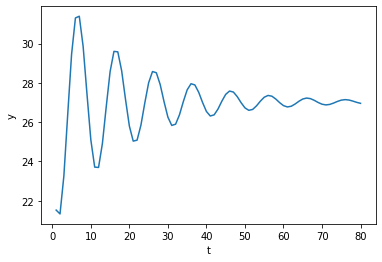If we set both initial values at the steady state value of $y_t$, namely,

$$y_{0} = y_{-1} = \frac{\alpha_{0}}{1 - \alpha_{1} - \alpha_{2}}$$

then $y_{t}$ will be constant

In :
y_1_steady = 𝛼0 / (1 - 𝛼1 - 𝛼2) # y_{-1}
y0_steady = 𝛼0 / (1 - 𝛼1 - 𝛼2)


In :
y_steady = A_inv @ b_steady

In :
plt.plot(np.arange(T)+1, y_steady)
plt.xlabel('t')
plt.ylabel('y')

plt.show()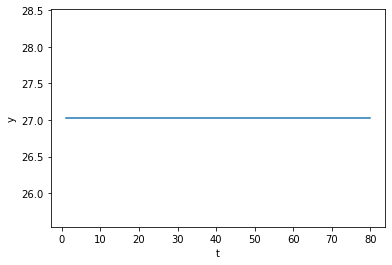To generate some excitement, we’ll follow in the spirit of the great economists Eugen Slusky and Ragnar Frisch and replace our original second-order difference equation with the following second-order stochastic linear difference equation:

$$y_{t} = \alpha_{0} + \alpha_{1} y_{t-1} + \alpha_{2} y_{t-2} + u_t \tag{2}$$

where $u_{t} \sim N\left(0, \sigma_{u}^{2}\right)$ and is IID, meaning independent and identically distributed.

We’ll stack these $T$ equations into a system cast in terms of matrix algebra.

Let’s define the random vector

$$u=\left[\begin{array}{c} u_{1}\\ u_{2}\\ \vdots\\ u_{T} \end{array}\right]$$

Where $A, b, y$ are defined as above, now assume that $y$ is governed by the system

$$A y = b + u$$

The solution for $y$ becomes

$$y = A^{-1} \left(b + u\right)$$

Let’s try it out in Python.

In :
𝜎u = 2.

In :
u = np.random.normal(0, 𝜎u, size=T)
y = A_inv @ (b + u)

In :
plt.plot(np.arange(T)+1, y)
plt.xlabel('t')
plt.ylabel('y')

plt.show()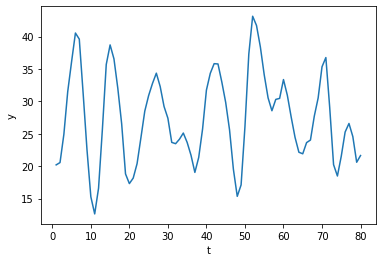The above time series looks a lot like (detrended) GDP series for a number of advanced countries in recent decades.

We can simulate $N$ paths.

In :
N = 100

for i in range(N):
u = np.random.normal(0, 𝜎u, size=T)
y = A_inv @ (b + u)
plt.plot(np.arange(T)+1, y, lw=0.5)

plt.xlabel('t')
plt.ylabel('y')

plt.show()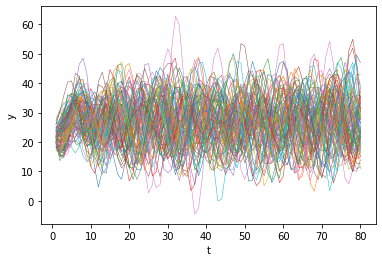Also consider the case when $y_{0}$ and $y_{-1}$ are at steady state.

In :
N = 100

for i in range(N):
u = np.random.normal(0, 𝜎u, size=T)

plt.xlabel('t')
plt.ylabel('y')

plt.show()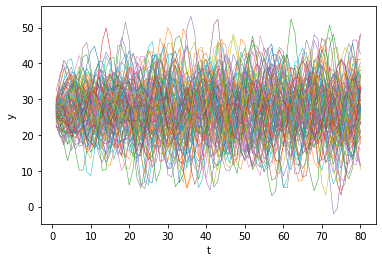## A forward looking model¶

Samuelson’s model is backwards looking in the sense that we give it initial conditions and let it run.

Let’s now turn to model that is forward looking.

We apply similar linear algebra machinery to study a perfect foresight model widely used as a benchmark in macroeconomics and finance.

As an example, we suppose that $p_t$ is the price of a stock and that $y_t$ is its dividend.

We assume that $y_t$ is determined by second-order difference equation that we analyzed just above, so that

$$y = A^{-1} \left(b + u\right)$$

Our perfect foresight model of stock prices is

$$p_{t} = \sum_{j=0}^{T-t} \beta^{j} y_{t+j}, \quad \beta \in (0,1)$$

where $\beta$ is a discount factor.

The model asserts that the price of the stock at $t$ equals the discounted present values of the (perfectly foreseen) future dividends.

Form

$$\underset{\equiv p}{\underbrace{\left[\begin{array}{c} p_{1}\\ p_{2}\\ p_{3}\\ \vdots\\ p_{T} \end{array}\right]}}=\underset{\equiv B}{\underbrace{\left[\begin{array}{ccccc} 1 & \beta & \beta^{2} & \cdots & \beta^{T-1}\\ 0 & 1 & \beta & \cdots & \beta^{T-2}\\ 0 & 0 & 1 & \cdots & \beta^{T-3}\\ \vdots & \vdots & \vdots & \vdots & \vdots\\ 0 & 0 & 0 & \cdots & 1 \end{array}\right]}}\left[\begin{array}{c} y_{1}\\ y_{2}\\ y_{3}\\ \vdots\\ y_{T} \end{array}\right]$$
In :
𝛽 = .96

In :
# construct B
B = np.zeros((T, T))

for i in range(T):
B[i, i:] = 𝛽 ** np.arange(0, T-i)

In :
B

Out:
array([[1.        , 0.96      , 0.9216    , ..., 0.04314048, 0.04141486,
0.03975826],
[0.        , 1.        , 0.96      , ..., 0.044938  , 0.04314048,
0.04141486],
[0.        , 0.        , 1.        , ..., 0.04681041, 0.044938  ,
0.04314048],
...,
[0.        , 0.        , 0.        , ..., 1.        , 0.96      ,
0.9216    ],
[0.        , 0.        , 0.        , ..., 0.        , 1.        ,
0.96      ],
[0.        , 0.        , 0.        , ..., 0.        , 0.        ,
1.        ]])
In :
𝜎u = 0.
u = np.random.normal(0, 𝜎u, size=T)
y = A_inv @ (b + u)

In :
p = B @ y

In :
plt.plot(np.arange(0, T)+1, y, label='y')
plt.plot(np.arange(0, T)+1, p, label='p')
plt.xlabel('t')
plt.ylabel('y/p')
plt.legend()

plt.show()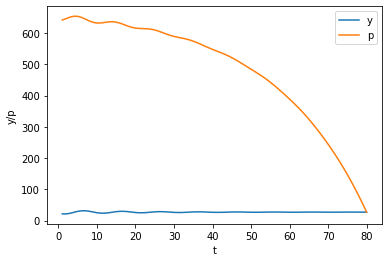Can you explain why the trend of the price is downward over time?

Also consider the case when $y_{0}$ and $y_{-1}$ are at the steady state.

In :
p_steady = B @ y_steady